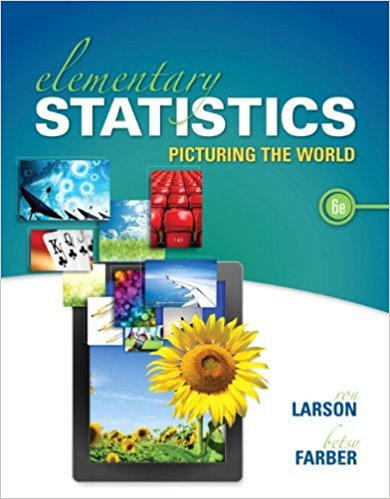×
×

# Solutions for Chapter 2.1: Frequency Distributions and Their Graphs## Full solutions for Elementary Statistics: Picturing the World | 6th Edition

ISBN: 9780321911216Solutions for Chapter 2.1: Frequency Distributions and Their Graphs

Solutions for Chapter 2.1
4 5 0 284 Reviews
17
3
##### ISBN: 9780321911216

This expansive textbook survival guide covers the following chapters and their solutions. Since 94 problems in chapter 2.1: Frequency Distributions and Their Graphs have been answered, more than 100594 students have viewed full step-by-step solutions from this chapter. Chapter 2.1: Frequency Distributions and Their Graphs includes 94 full step-by-step solutions. This textbook survival guide was created for the textbook: Elementary Statistics: Picturing the World , edition: 6. Elementary Statistics: Picturing the World was written by and is associated to the ISBN: 9780321911216.

Key Statistics Terms and definitions covered in this textbook

A formula used to determine the probability of the union of two (or more) events from the probabilities of the events and their intersection(s).

• Attribute control chart

Any control chart for a discrete random variable. See Variables control chart.

• Bimodal distribution.

A distribution with two modes

• Binomial random variable

A discrete random variable that equals the number of successes in a ixed number of Bernoulli trials.

• Conditional probability distribution

The distribution of a random variable given that the random experiment produces an outcome in an event. The given event might specify values for one or more other random variables

• Consistent estimator

An estimator that converges in probability to the true value of the estimated parameter as the sample size increases.

• Continuous random variable.

A random variable with an interval (either inite or ininite) of real numbers for its range.

• Counting techniques

Formulas used to determine the number of elements in sample spaces and events.

• Defect concentration diagram

A quality tool that graphically shows the location of defects on a part or in a process.

• Designed experiment

An experiment in which the tests are planned in advance and the plans usually incorporate statistical models. See Experiment

• Distribution function

Another name for a cumulative distribution function.

• Error sum of squares

In analysis of variance, this is the portion of total variability that is due to the random component in the data. It is usually based on replication of observations at certain treatment combinations in the experiment. It is sometimes called the residual sum of squares, although this is really a better term to use only when the sum of squares is based on the remnants of a model-itting process and not on replication.

• Estimator (or point estimator)

A procedure for producing an estimate of a parameter of interest. An estimator is usually a function of only sample data values, and when these data values are available, it results in an estimate of the parameter of interest.

• Exhaustive

A property of a collection of events that indicates that their union equals the sample space.

• Extra sum of squares method

A method used in regression analysis to conduct a hypothesis test for the additional contribution of one or more variables to a model.

• Factorial experiment

A type of experimental design in which every level of one factor is tested in combination with every level of another factor. In general, in a factorial experiment, all possible combinations of factor levels are tested.

• False alarm

A signal from a control chart when no assignable causes are present

• Fractional factorial experiment

A type of factorial experiment in which not all possible treatment combinations are run. This is usually done to reduce the size of an experiment with several factors.

• Gamma function

A function used in the probability density function of a gamma random variable that can be considered to extend factorials

• Generating function

A function that is used to determine properties of the probability distribution of a random variable. See Moment-generating function

×